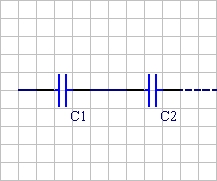# Capacitor Combination Calculator

## Capacitors Combined in Parallel and Series Explained.

Along with resistors and inductors, capacitors are one of the most fundamental passive components used in any circuit design. You would have to look very hard to find a circuit which didn't have a capacitor in it. Not only can capacitors be use in singular, they can be combined in parallel or in series.

When capacitors are hooked up in parallel the capacitor farad values add together but the maximum voltage will be that of the lowest rated capacitor.
Note: In your circuit designs always allow a 50% or better safety margin for the maximum voltage of capacitors. For example, if the voltage of your circuit is 5 volts, then your capacitors should be rated for at least 10 volts.Enter a value for C1 and C2then click Calculate. C1 uF   +   C2 uFCt   =   0 uFCapacitors hooked up in series combine as reciprocals. To get the reciprocal of a number (except zero has no reciprocal), just divide 1 by the number.
Note: As shown by the equation below, capacitors in series only hold a fraction of their individual capacitance!=    0.0000 uFSo when does it make sense to put capacitors in series? Since you get less capacitance and less charge storage than with either alone, why do it? Series capacitors are sometimes done in electronics design because capacitors have a maximum working voltage. Capacitors hooked up in series will increase the maximum voltage. For example, having two 630 volt capacitors in series, will increase the working voltage to 1260 volts!